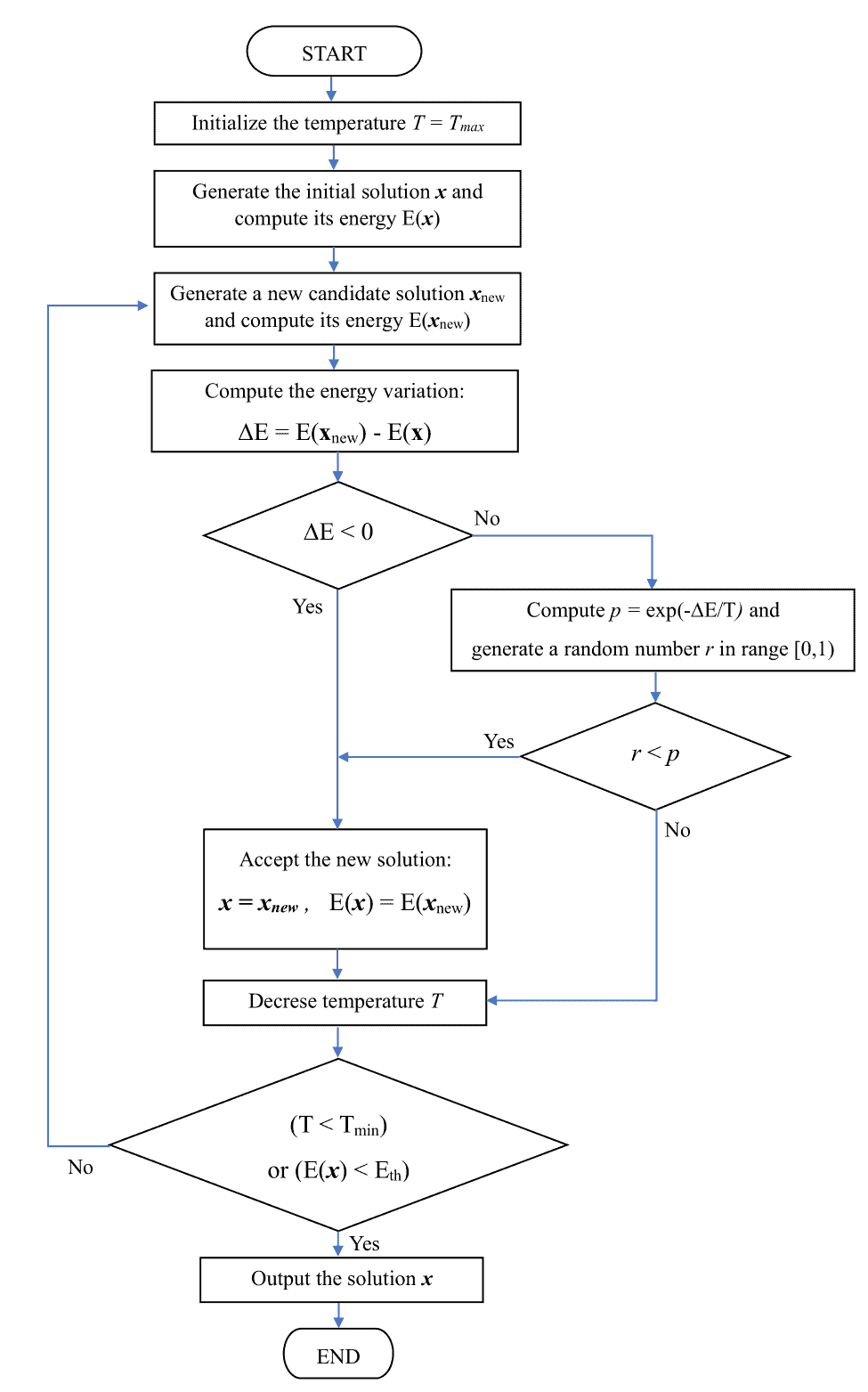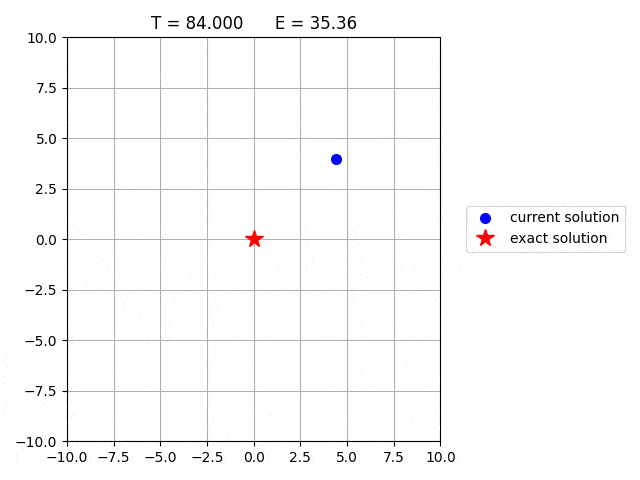## 1. Overview

In this tutorial, we’ll review the Simulated Annealing (SA), a metaheuristic algorithm commonly used for optimization problems with large search spaces. Then, we illustrate the SA optimization procedure and show how to minimize a function.

## 2. The Physics Behind the Algorithm

SA is a metaheuristic optimization technique introduced by Kirkpatrick et al. in 1983 to solve the Travelling Salesman Problem (TSP).

The SA algorithm is based on the annealing process used in metallurgy, where a metal is heated to a high temperature quickly and then gradually cooled. At high temperatures, the atoms move fast, and when the temperature is reduced, their kinetic energy decreases as well. At the end of the annealing process, the atoms fall into a more ordered state, and the material is more ductile and easier to work with.

Similarly, in SA, a search process starts with a high-energy state (an initial solution) and gradually lowers the temperature (a control parameter) until it reaches a state of minimum energy (the optimal solution).

SA has been successfully applied to a wide range of optimization problems, such as TSP, protein folding, graph partitioning, and job-shop scheduling. The main advantage of SA is its ability to escape from local minima and converge to a global minimum. SA is also relatively easy to implement and does not require a priori knowledge of the search space.

## 3. Algorithm

The simulated annealing process starts with an initial solution and then iteratively improves the current solution by randomly perturbing it and accepting the perturbation with a certain probability. The probability of accepting a worse solution is initially high and gradually decreases as the number of iterations increases.

The SA algorithm is quite simple, and it can be straightforwardly implemented as described below.

### 3.1. Define the Problem

First, we need to define the problem to optimize. This involves defining the energy function, i.e., the function to minimize or maximize. For example, if we want to minimize a real-valued function of two variables, e.g.,, the energy corresponds to the functionitself. In the case of the TSP, the energy related to a sequence of cities is represented by the total length of the travel.

Once the energy function is defined, we need to set the initial temperature value and the initial candidate solution. The latter can be generated randomly or using some other heuristic method. Then we compute the energy of the initial candidate solution.

### 3.2. Define the Perturbation Function

A perturbation function is defined to generate new candidate solutions. This function should generate solutions that are close to the current solution but not too similar. For example, if we want to minimize a function, we can randomly perturb the current solution by adding a random value between -0.1 and 0.1 to bothand. In the case of the TSP, a new candidate solution can be generated by swapping two cities in the traveling order of the current solution.

### 3.3. Acceptance Criterion

The acceptance criterion determines whether a new solution is accepted or rejected. The acceptance depends on the energy difference between the new solution and the current solution, as well as the current temperature. The classic acceptance criterion of SA comes from statistical mechanics, and it is based on the Boltzmann probability distribution. A system in thermal equilibrium at temperaturecan be found in a state with energywith a probability proportional to(1)whereis the Boltzmann constant. Hence, at low temperatures, there is a small chance that the system is in a high-energy state. This plays a crucial role in SA because an increase in energy allows escape from local minima and find the global minimum.

Based on the Boltzmann distribution, the following algorithm defines the criterion for accepting an energy variationat temperature.A candidate solution with lower energy is always accepted. Conversely, a candidate solution with higher energy is accepted randomly with probability(for our purpose, we can set). The latter case can be implemented by comparing the probability with a random value generated in the range.

### 3.4. Temperature Schedule

The temperature schedule determines how the temperature of the system changes over time. In the beginning, the temperature is high so that the algorithm can explore a wide range of solutions, even if they are worse than the current solution. As the iterations increase, the temperature gradually decreases, so the algorithm becomes more selective and accepts better solutions with higher probability. A simple scheduling can be obtained by dividing the current temperature by a factor, which is less than 1.

### 3.5. Run the SA Algorithm

Finally, run the algorithm by iteratively applying the perturbation function and acceptance criterion to the current solution. The algorithm terminates when the temperature has cooled to a certain levelor when the energy of the current solution is lower than a fixed threshold.

Here’s the pseudocode of SA:## 4. SA Flowchart

Here, we provide a detailed flowchart representing all steps of SA:## 5. Example

To better understand the algorithm, we use SA to illustrate the minimization of the function. We used as search space a grid of size 101101 placed in the square area defined by. We set the cooling rateand the initial solution. At each step, a new solution is generated by randomly shifting the current solution byinanddirection.

Here’s an animation showing the candidate solution, its energy, and the temperature at each step:We can observe that worse solutions are frequently accepted when the temperature is high. Conversely, when the temperature is low (e.g.,), the algorithm is more selective, and better solutions are accepted with higher probability.

## 6. Conclusion

In this article, we provided an overview of the SA algorithm. We illustrated the optimization procedure and provided a practical example of its application.